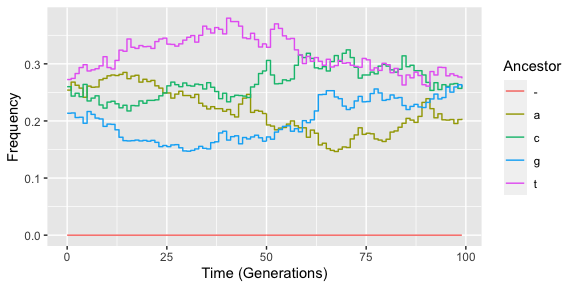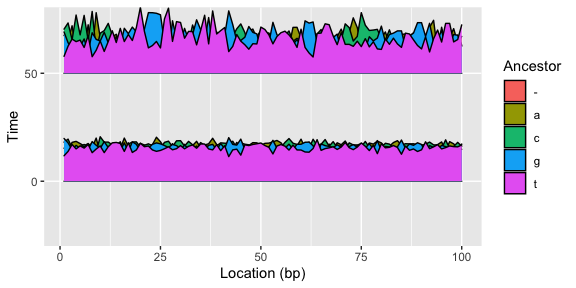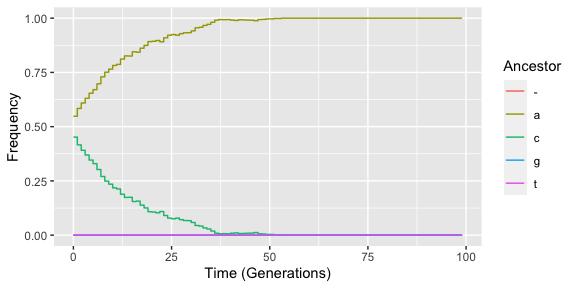# Empirical data

## Empirical data

By default, the package uses local ancestry data, where each locus can be uniquely traced back to one of the founding populations of the admixed focal population. If, however, you are more interested in admixing sequence data, the GenomeAdmixR package provides this optionality as well, where you can import your own sequences and admix those in a similar fashion.

  fake_input_data <-
marker_locations = 1:10)

fake_input_data$markers ##  1 2 3 4 5 6 7 8 9 10  fake_input_data$genomes
##       [,1] [,2] [,3] [,4] [,5] [,6] [,7] [,8] [,9] [,10]
##  [1,]    3    4    2    2    3    2    1    2    4     4
##  [2,]    3    2    3    1    3    4    2    3    1     1
##  [3,]    3    3    2    4    4    1    1    1    4     2
##  [4,]    1    2    4    1    3    3    3    3    4     2
##  [5,]    3    3    1    1    4    3    3    4    2     2
##  [6,]    4    2    4    3    3    3    2    2    4     3
##  [7,]    1    1    1    3    3    1    4    3    4     1
##  [8,]    3    2    4    3    4    2    3    3    4     2
##  [9,]    2    3    3    4    2    2    3    1    3     4
## [10,]    3    3    3    4    4    3    4    4    2     1
## [11,]    2    1    2    4    4    2    2    1    4     3
## [12,]    2    3    3    1    3    2    2    2    2     2
## [13,]    3    4    4    3    4    2    2    2    1     2
## [14,]    3    4    2    2    3    4    2    1    4     3
## [15,]    3    2    1    1    3    1    4    3    2     1
## [16,]    1    2    4    3    3    3    4    3    3     4
## [17,]    3    2    4    1    1    2    3    4    4     4
## [18,]    4    2    4    3    1    3    1    2    4     2
## [19,]    1    3    2    4    2    1    2    3    1     3
## [20,]    2    4    3    3    2    2    4    3    2     3

Here, we create fake input data in absence of an example file. The data consists of two components: a genome matrix and a vector with marker locations. The genome matrix has two rows per individual (one for each chromosome) and one column per molecular marker. The number of columns of the genome matrix should match the length of the marker vector. Instead of using a/c/t/g, we use numerical notation, e.g. 1/2/3/4 (with missing data = 0). Instead, you can also load sequence data from a VCF file (function ) or from PLINK style data (function ). The loaded data can now be used as input for a simulation using the sequence_module:

simulated_pop <- simulate_admixture(
module = sequence_module(molecular_data = fake_input_data),
pop_size = 100,
total_runtime = 100)

If you want to study admixture, you might have VCF data with individuals from multiple populations. GenomeAdmixR provides functionality to use this data as seeding data as well, although we do ask you to prepare two distinct VCF files, where each VCF contains the individuals from each population. Because only accepts a single input matrix, we can artifically create an initial hybrid input swarm by loading data from multiple VCF files, and then randomly sampling individuals from these datasets in order to form the starting dataset. We can do so using the function combine_input_data:

  chosen_markers <- 1:100
fake_input_data1 <-
marker_locations = chosen_markers,
used_nucleotides = 1:2)
fake_input_data2 <-
marker_locations = chosen_markers,
used_nucleotides = 3:4)

combined_data <- combine_input_data(input_data_list = list(fake_input_data1,
fake_input_data2),
frequencies = c(0.5, 0.5),
pop_size = 1000)

We can use this input data as a starting point for a simulation again:

simulated_pop <- simulate_admixture(
module = sequence_module(molecular_data = combined_data,
markers = 1:100,
morgan = 1),
pop_size = 1000,
total_runtime = 100)

We have simulated here a population of 1000 individuals (initially randomly drawn from the sequences we created using combine_input_data), for 100 generations. The result is identical in structure to results from ‘simulate_admixture’ and we can use the same visualisation and analytical devices:

plot_over_time(simulated_pop$frequencies, focal_location = 50)plot_joyplot_frequencies(simulated_pop$frequencies, time_points = c(0, 50, 100))Furthermore, we can also perform selection on a certain allele, where the location under selection (first entry in the selection matrix) has to exist in the original dataset.

selection_matrix <- matrix(NA, nrow = 1, ncol = 5)
selection_matrix[1, ] <- c(50, 1, 1.1, 1.2, 1)

# we expect allele 'a' to do well at 0.5 Morgan:
module = sequence_module(molecular_data = fake_input_data1,
morgan = 1,
markers = chosen_markers),
pop_size = 1000,
total_runtime = 100,
select_matrix = selection_matrix)
## Found a selection matrix, performing simulation including selection
plot_over_time(selected_pop\$frequencies, focal_location = 50)# Migration

Sequence data can also be used to seed a simulation where two populations exchange migrants, by specifying parameters for the migration argument, using the migration_settings function:

migr_pop <-
module = sequence_module(molecular_data = list(fake_input_data1,
fake_input_data2)),
migration = migration_settings(migration_rate = 0.01,
population_size = c(100, 100)),
total_runtime = 100)

# Using simulation data as input

Lastly, not only “true” sequencing data can be used as input, but also previous output from GenomeAdmixR simulations. In order to so, output from previous simulations can be converted to genomeadmixr_data, either using pre-existing molecular markers as used in the simulation, or by superimposing markers on new locations.

simulated_pop <- simulate_admixture(
module = ancestry_module(),
pop_size = 100,
total_runtime = 100)
prepared_pop  <-
markers = seq(0, 1, length.out = 100))

module = sequence_module(molecular_data = prepared_pop),
pop_size = 100,
total_runtime = 100)

# Mutation rate

When using sequencing data, the simulations can also be extended using mutation. One can do so by specifying the mutation rate (in probability per bp per generation), and by specifying the substitution matrix, where the substitution matrix is a 4x4 matrix, that specifies the probability of the different substitutions and transversions. For instance, for the JC69 model, the substitution matrix is given by:

writeLines(print_mat(matrix(1 / 4, nrow = 4, ncol = 4)))
$\begin{bmatrix} 0.25 & 0.25 & 0.25 & 0.25 \\ 0.25 & 0.25 & 0.25 & 0.25 \\ 0.25 & 0.25 & 0.25 & 0.25 \\ 0.25 & 0.25 & 0.25 & 0.25 \end{bmatrix}$

Please note that this is not necessarily the rate matrix! In the substitution matrix we use, all rows MUST sum to 1 (as these represent the probabilities of mutating into another base). For instance, for the K80 model with parameter kappa, we would get the following substitution matrix, with kappa = 0.2:

kappa <- 0.2
diag_entry <- 1 - (1 / 4 + 1 / 4 + 0.2 / 4)

writeLines(print_mat(matrix(c(diag_entry, kappa, 1 / 4, 1 / 4,
kappa, diag_entry, 1 / 4, 1 / 4,
1 / 4, 1 / 4, diag_entry, kappa,
1 / 4, 1 / 4, kappa, diag_entry),
nrow = 4, ncol = 4)))
$\begin{bmatrix} 0.45 & 0.2 & 0.25 & 0.25 \\ 0.2 & 0.45 & 0.25 & 0.25 \\ 0.25 & 0.25 & 0.45 & 0.2 \\ 0.25 & 0.25 & 0.2 & 0.45 \end{bmatrix}$

We understand that it might be tricky to make these calculations a priori, therefore we automatically translate the substitution matrix to the probability matrix required in case the matrix is a rate matrix. Thus, summarising, the substitution matrix provides the conditional probability of mutating to another base, given that mutation occurs (as governed by the overall mutation rate). Using artificial sequencing data, we can for instance show how new bases arise in the population due to mutation:

  fake_input_data3 <-
marker_locations = 1:1000,
used_nucleotides = 1)

kappa <- 0.5
rate_matrix <- matrix(c(0, kappa, 1, 1,
kappa, 0, 1, 1,
1, 1, 0, kappa,
1, 1, kappa, 0), nrow = 4, ncol = 4)

mutation_rate <- 1e-5

module = sequence_module(molecular_data = fake_input_data3,
morgan = 1,
markers = chosen_markers,
mutation_rate = mutation_rate,
substitution_matrix = rate_matrix),
pop_size = 1000,
total_runtime = 100)
## Warning in verify_substitution_matrix(substitution_matrix): found rate matrix,
## rescaled all entries
  freqs <- calculate_allele_frequencies(simulated_pop)

require(magrittr, quietly = TRUE)
freqs %>%
dplyr::group_by(ancestor) %>%
dplyr::summarise(mean(frequency))
## # A tibble: 4 × 2
##   ancestor mean(frequency)
##   <chr>                <dbl>
## 1 a                        1
## 2 c                        0
## 3 g                        0
## 4 t                        0

The final results show that indeed mutations have arisen in the population: the initial fake data only contained ‘a’ for all sequences, whereas at the end of the simulation, we see that the other bases also exist in non-zero frequencies.